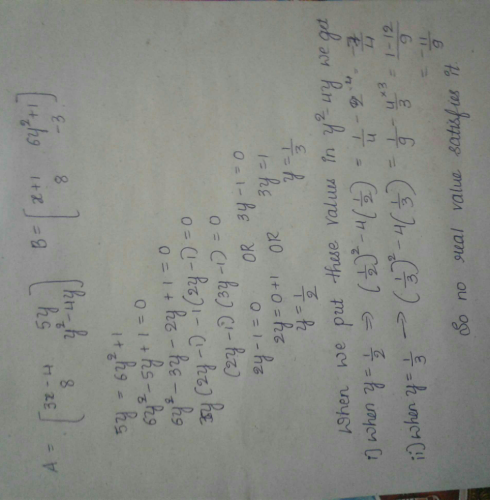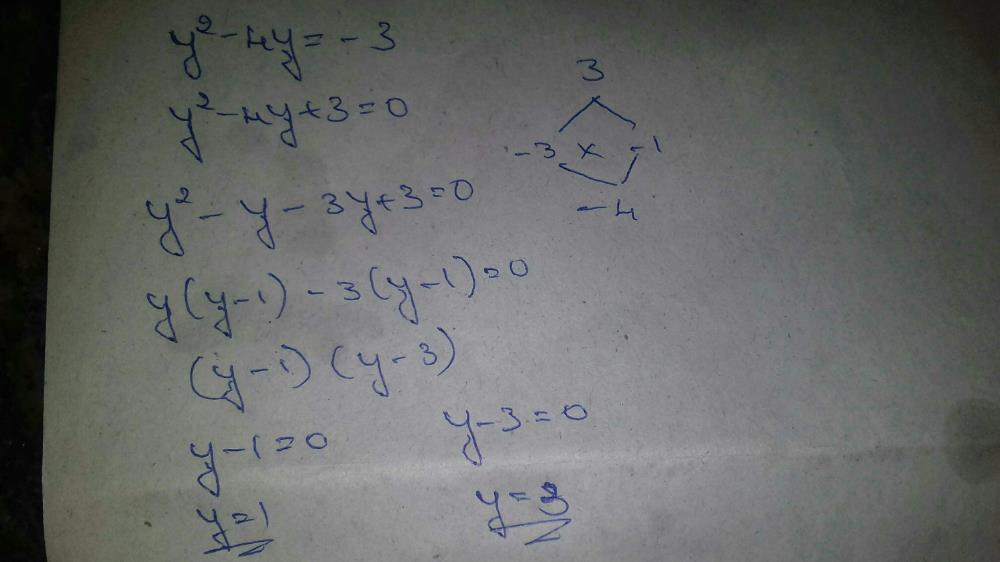Courses

# For what real value of y will matrix A be equal to... more matrix B, where​a)1, 3b)No real valuec)1/3, 1/2d)2 and 3Correct answer is option 'c'. Can you explain this answer? Related Test: Test: Introduction To Matrices

## JEE Question

By Sanjay Kumar · Jun 05, 2018 ·JEE
Sanjay Kumar answered May 30, 2018
y^2-4y+3=0 (y-1)(y-3)=0 y=1,3Hint:-solve 5y=6y^2+1 & y^2-4y=-3

Shikha Sony answered Dec 20, 2018
As both matrix are eaual given so each element are equal y^2-4y=-3
y^2 -3y-y+3=0
y(y-3)-1(y-3)=0
y-1=0, y-3=0
y=1,3 similarly on solving other quadratic equation on y we get
y=1/3,1/2
no common value so no real value

Shamith Sourab answered Mar 26, 2019Naincy Tripathi answered Jun 06, 2018
(5y = 6y^2+1) (6y^2 - 5y +1 = 0) apply shri dharacharya method nd solve u will get 1/2 nd1/3 which is not real HENCE OPTION b IS CORRECT

⇒ y2 - 4y = -3
⇒ y2 - 4y + 3 = 0
⇒ y2 -3y - y +3 = 0
⇒ y (y - 3) -1 (y-3) = 0
⇒(y - 1) (y - 3) = 0
⇒ y = 1,3
But these are not real numbers.
We have another equation:
⇒ 5y = 6y2 + 1
⇒ 6y2 -5y +1 = 0
⇒ 6y2 -3y - 2y + 1 = 0
⇒ 3y (2y - 1) - 1 (2y - 1) = 0
⇒ (3y - 1) (2y - 1) = 0
⇒ y = 1/2, 1/3
Hence value of y is 1/2, 1/3

Y must satisfy both conditions 5y=6y(square)+1y(square)-4y=-3Which is not possible to have real number that's why answer is option B

Gudiya Jain answered Mar 23, 2020
Comparing the elements of matrices A and B, we get two different sets of values of y, where nothing is common. So, we can say that no real values exist satisfying the given matrices. Note that it doesn't mean that values obtained are not real.

Rishu Kumar answered Feb 20, 2019
Y2-4y=-3 on solving we get y=1,3
6y2-5y+1=0 on solving we get y=1/3,1/2
there is no common value so y is not real .

Jeghan Sankar answered Jul 03, 2018

Amar Kumar answered May 20, 2020
No real value

Zain Khan answered Feb 11, 2019
Consider y*y-4y =-3

y*y-4y+3=0

factors are -1,-3
=> y-3=0 ; y=3
y-1=0; y=1
a)

Amaan Ansari answered Jan 30, 2019
Yes no real value should be correct answer

Gourav Mandal answered Sep 19, 2019
Why

Dev Garwa answered Oct 09, 2019
How 1 and 3 are not real numbers?